### Linear Assignment (Matching)

The linear assignment problem (LAP) is a fundamental problem in combinatorial optimization that involves assigning workers to tasks at minimal costs. In graph theoretic terms, LAP is equivalent to finding a minimum-weight matching in a weighted bipartite graph. In a bipartite graph, the nodes can be divided into two disjoint sets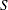(workers) and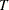(tasks) such that every link connects a node into a node in. That is, the node setsandare independent. The concept of assigning workers to tasks can be generalized to the assignment of any abstract object from one group to some abstract object from a second group.

The linear assignment problem can be formulated as an integer programming optimization problem. The form of the problem depends on the sizes of the two input sets,and. Let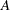represent the set of possible assignments between setsand. In the bipartite graph, these are the links. If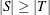, then the following optimization problem is solved: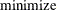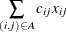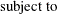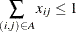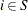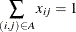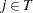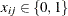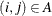This model allows for some elements of set(workers) to go unassigned (if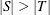). However, if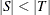, then the following optimization problem is solved:This model allows for some elements of set(tasks) to go unassigned.

In PROC OPTNET, the linear assignment problem solver can be invoked by using the LINEAR_ASSIGNMENT statement. The options for this statement are described in the section LINEAR_ASSIGNMENT Statement. The algorithm used in PROC OPTNET for solving LAP is based on augmentation of shortest paths (Jonker and Volgenant 1987). This algorithm can be applied to either matrix data input (see the section Matrix Input Data) or graph data input (see the section Graph Input Data) as long as the graph is bipartite.

The resulting assignment (or matching) is given in the output data set that is specified in the OUT= option in the LINEAR_ASSIGNMENT statement.